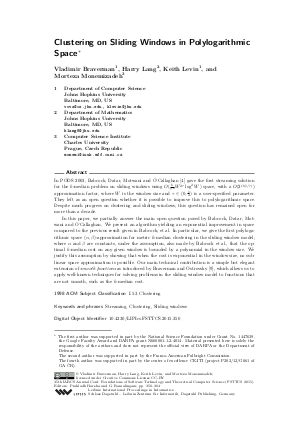Document# Clustering on Sliding Windows in Polylogarithmic Space## File

LIPIcs.FSTTCS.2015.350.pdf
• Filesize: 0.55 MB
• 15 pages

## Cite As

Vladimir Braverman, Harry Lang, Keith Levin, and Morteza Monemizadeh. Clustering on Sliding Windows in Polylogarithmic Space. In 35th IARCS Annual Conference on Foundations of Software Technology and Theoretical Computer Science (FSTTCS 2015). Leibniz International Proceedings in Informatics (LIPIcs), Volume 45, pp. 350-364, Schloss Dagstuhl - Leibniz-Zentrum für Informatik (2015)
https://doi.org/10.4230/LIPIcs.FSTTCS.2015.350

## Abstract

In PODS 2003, Babcock, Datar, Motwani and O'Callaghan gave the first streaming solution for the k-median problem on sliding windows using O(frack k tau^4 W^2tau log^2 W) space, with a O(2^O(1/tau)) approximation factor, where W is the window size and tau in (0,1/2) is a user-specified parameter. They left as an open question whether it is possible to improve this to polylogarithmic space. Despite much progress on clustering and sliding windows, this question has remained open for more than a decade. In this paper, we partially answer the main open question posed by Babcock, Datar, Motwani and O'Callaghan. We present an algorithm yielding an exponential improvement in space compared to the previous result given in Babcock, et al. In particular, we give the first polylogarithmic space (alpha,beta)-approximation for metric k-median clustering in the sliding window model, where alpha and beta are constants, under the assumption, also made by Babcock et al., that the optimal k-median cost on any given window is bounded by a polynomial in the window size. We justify this assumption by showing that when the cost is exponential in the window size, no sublinear space approximation is possible. Our main technical contribution is a simple but elegant extension of smooth functions as introduced by Braverman and Ostrovsky, which allows us to apply well-known techniques for solving problems in the sliding window model to functions that are not smooth, such as the k-median cost.
##### Keywords
• Streaming
• Clustering
• Sliding windows

## Metrics

• Access Statistics
• Total Accesses (updated on a weekly basis)
0